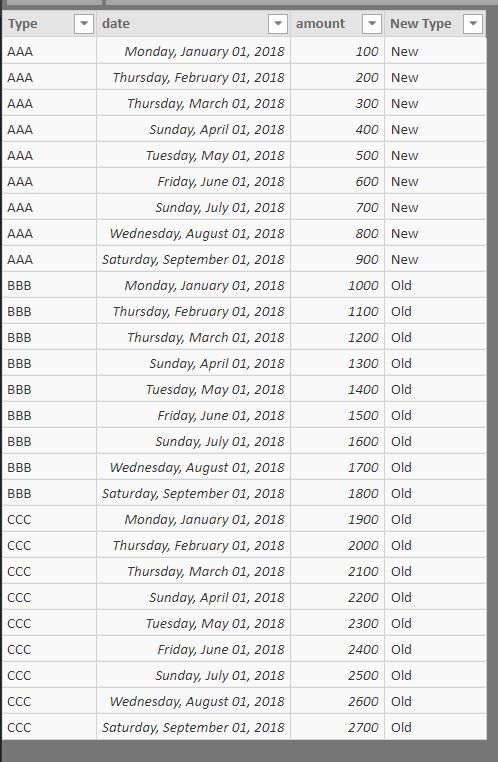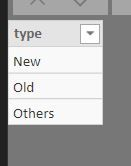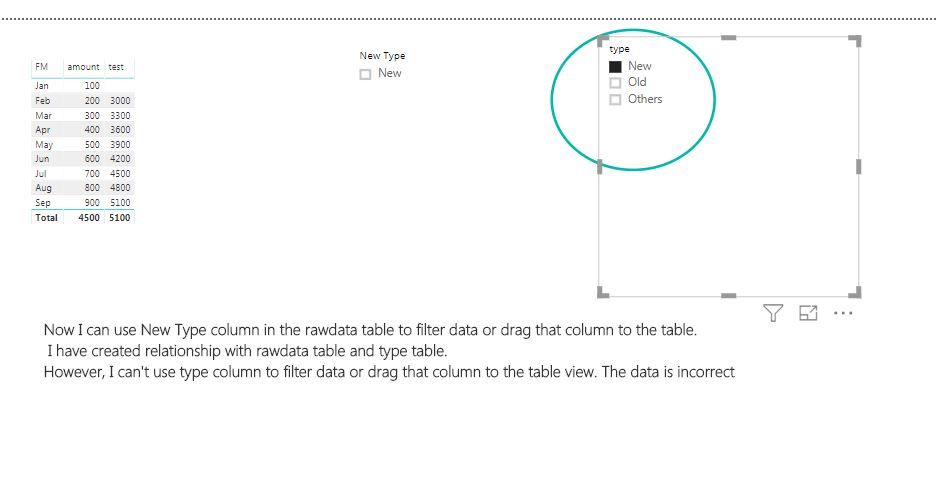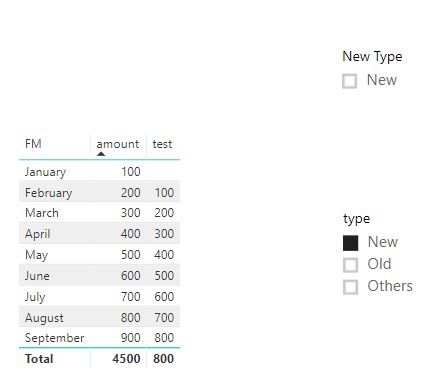cancel
Showing results for
Did you mean:Super User

## URGENT! How to filter data in different table?

Hi all,

I have three tables: rawdata, date and type. In the rawdata, I added a new column whose name is "New Type". Then I linked the "New Type" column to the "type" column in type table.

I want to calculate the last month's amount and the last second month's amount. I know we can use dateadd formula, but that formula does not work when you choose several month and display data as a card.

Below is the formula I used:

test =
VAR maxdate=MAX('Rawdata'[date])
VAR PM1 = DATE(YEAR(maxdate),MONTH(maxdate)-1,01)
VAR totalamount=sum(Rawdata[amount])
VAR PM1TOTAL=CALCULATE([totalamount],FILTER(ALLEXCEPT(Rawdata,Rawdata[New Type]),'Rawdata'[date]=PM1))
RETURN
PM1TOTAL

Then I can use New Type column to filter data and drag that column to a table view. However, when I use type column to filter, the data is incoorect.

Can anyone help me on that?

Date table:Rawdata table(New Type column was created in PBI,   New Type = SWITCH('Rawdata'[Type],"AAA","New","Old")Type tableI got the wrong data when I use type column to filter, (test column should show last month's amount)Thanks a lot

Proud to be a Super User!

1 ACCEPTED SOLUTIONCommunity Support

hi, @ryan_mayu

```test =
VAR maxdate=MAX('Rawdata'[date])
VAR PM1 = DATE(YEAR(maxdate),MONTH(maxdate)-1,01)
VAR totalamount=sum(Rawdata[amount])
VAR PM1TOTAL=CALCULATE([totalamount],FILTER(ALLEXCEPT(Rawdata,Rawdata[New Type],'Type table'[type]),'Rawdata'[date]=PM1))
RETURN
PM1TOTAL```

In your conditional, ALLEXCEPT(Rawdata,Rawdata[New Type]) , so only Rawdata[New Type] can filter the data.

you just add a conditional for 'Type table'[type] in ALLEXCEPT(Rawdata,Rawdata[New Type],'Type table'[type])

Result:Best Regards,

Lin

Community Support Team _ Lin
If this post helps, then please consider Accept it as the solution to help the other members find it more quickly.
4 REPLIES 4Super User

Can anyone help me on this? It's really urgent. Thanks in advance

Proud to be a Super User!Community Support

hi, @ryan_mayu

```test =
VAR maxdate=MAX('Rawdata'[date])
VAR PM1 = DATE(YEAR(maxdate),MONTH(maxdate)-1,01)
VAR totalamount=sum(Rawdata[amount])
VAR PM1TOTAL=CALCULATE([totalamount],FILTER(ALLEXCEPT(Rawdata,Rawdata[New Type],'Type table'[type]),'Rawdata'[date]=PM1))
RETURN
PM1TOTAL```

In your conditional, ALLEXCEPT(Rawdata,Rawdata[New Type]) , so only Rawdata[New Type] can filter the data.

you just add a conditional for 'Type table'[type] in ALLEXCEPT(Rawdata,Rawdata[New Type],'Type table'[type])

Result:Best Regards,

Lin

Community Support Team _ Lin
If this post helps, then please consider Accept it as the solution to help the other members find it more quickly.Super User

Hi Lin,

It works. Many thanks for your help. Really apprecated that.

Proud to be a Super User!Super UserProud to be a Super User!